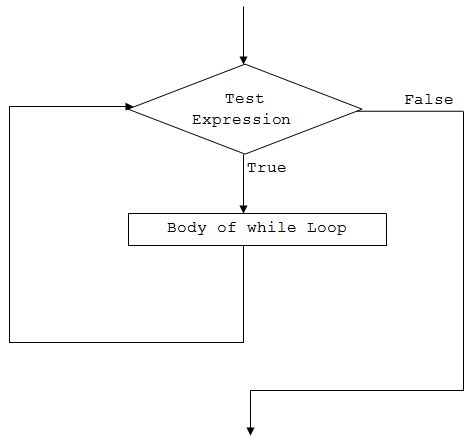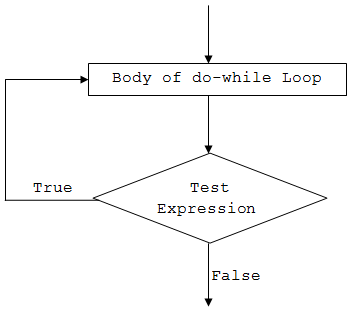# C# while and do...while loop

In this article, we will learn about while and do...while loop in C#, how to use them and difference between them.

In programming, it is often desired to execute certain block of statements for a specified number of times. A possible solution will be to type those statements for the required number of times. However, the number of repetition may not be known in advance (during compile time) or maybe large enough (say 10000).

The best solution to such problem is loop. Loops are used in programming to repeatedly execute a certain block of statements until some condition is met.

In this article, we'll learn to use while loops in C#.

## C# while loop

The while keyword is used to create while loop in C#. The syntax for while loop is:

```while (test-expression)
{
// body of while
}
```

### How while loop works?

1. C# while loop consists of a `test-expression`.
2. If the `test-expression` is evaluated to `true`,
1. statements inside the while loop are executed.
2. after execution, the `test-expression` is evaluated again.
3. If the `test-expression` is evaluated to `false`, the while loop terminates.

### while loop FlowchartWorking of C# while loop

### Example 1: while Loop

``````using System;

namespace Loop
{
class WhileLoop
{
public static void Main(string[] args)
{
int i=1;
while (i<=5)
{
Console.WriteLine("C# For Loop: Iteration {0}", i);
i++;
}
}
}
}
``````

When we run the program, the output will be:

```C# For Loop: Iteration 1
C# For Loop: Iteration 2
C# For Loop: Iteration 3
C# For Loop: Iteration 4
C# For Loop: Iteration 5```

Initially the value of i is 1.

When the program reaches the while loop statement,

• the test expression `i <=5` is evaluated. Since i is 1 and `1 <= 5` is `true`, it executes the body of the while loop. Here, the line is printed on the screen with Iteration 1, and the value of i is increased by 1 to become 2.
• Now, the test expression (`i <=5`) is evaluated again. This time too, the expression returns `true` (2 <= 5), so the line is printed on the screen and the value of i is now incremented to 3..
• This goes and the while loop executes until i becomes 6. At this point, the test-expression will evaluate to `false` and hence the loop terminates.

### Example 2: while loop to compute sum of first 5 natural numbers

``````using System;

namespace Loop
{
class WhileLoop
{
public static void Main(string[] args)
{
int i=1, sum=0;

while (i<=5)
{
sum += i;
i++;
}
Console.WriteLine("Sum = {0}", sum);
}
}
}
``````

When we run the program, the output will be:

`Sum = 15`

This program computes the sum of first 5 natural numbers.

• Initially the value of sum is initialized to 0.
• On each iteration, the value of sum is updated to `sum+i` and the value of i is incremented by 1.
• When the value of i reaches 6, the test expression `i<=5` will return false and the loop terminates.

Let's see what happens in the given program on each iteration.

Initially, i = 1, sum = 0

While loop execution steps
Iteration Value of i i<=5 Value of sum
1 1 true 0+1 = 1
2 2 true 1+2 = 3
3 3 true 3+3 = 6
4 4 true 6+4 = 10
5 5 true 10+5 = 15
6 6 false Loop terminates

So, the final value of sum will be 15.

## C# do...while loop

The do and while keyword is used to create a do...while loop. It is similar to a while loop, however there is a major difference between them.

In while loop, the condition is checked before the body is executed. It is the exact opposite in do...while loop, i.e. condition is checked after the body is executed.

This is why, the body of do...while loop will execute at least once irrespective to the test-expression.

The syntax for do...while loop is:

```do
{
// body of do while loop
} while (test-expression);
```

### How do...while loop works?

1. The body of do...while loop is executed at first.
2. Then the `test-expression` is evaluated.
3. If the `test-expression` is `true`, the body of loop is executed.
4. When the `test-expression` is `false`, do...while loop terminates.

### do...while loop FlowchartWorking of C# do...while loop

### Example 3: do...while loop

``````using System;

namespace Loop
{
class DoWhileLoop
{
public static void Main(string[] args)
{
int i = 1, n = 5, product;

do
{
product = n * i;
Console.WriteLine("{0} * {1} = {2}", n, i, product);
i++;
} while (i <= 10);
}
}
}
``````

When we run the program, the output will be:

```5 * 1 = 5
5 * 2 = 10
5 * 3 = 15
5 * 4 = 20
5 * 5 = 25
5 * 6 = 30
5 * 7 = 35
5 * 8 = 40
5 * 9 = 45
5 * 10 = 50```

As we can see, the above program prints the multiplication table of a number (5).

• Initially, the value of i is 1. The program, then enters the body of do..while loop without checking any condition (as opposed to while loop).
• Inside the body, product is calculated and printed on the screen. The value of i is then incremented to 2.
• After the execution of the loop’s body, the test expression `i <= 10` is evaluated. In total, the do...while loop will run for 10 times.
• Finally, when the value of i is 11, the test-expression evaluates to `false` and hence terminates the loop.

## Infinite while and do...while loop

If the test expression in the while and do...while loop never evaluates to `false`, the body of loop will run forever. Such loops are called infinite loop.

For example:

### Infinite while loop

```while (true)
{
// body of while loop
}
```

### Infinite do...while loop

```do
{
// body of while loop
} while (true);
```

The infinite loop is useful when we need a loop to run as long as our program runs.

For example, if your program is an animation, you will need to constantly run it until it is stopped. In such cases, an infinite loop is necessary to keep running the animation repeatedly.

Did you find this article helpful?